# Momentum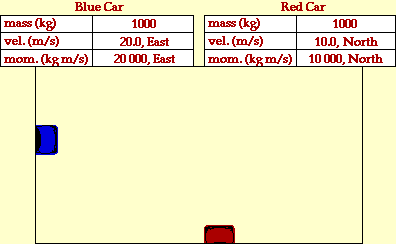• The momentum of a particle is defined as the product of its mass times its velocity. It is a vector quantity.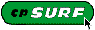Hewitt's CP Surf Unit I: Mechanics  Unit 1: Chapter 7 Learning Links

## Conservation of Momentum

The momentum of an isolated system is a constant. The vector sum of the momenta mv of all the objects of a system cannot be changed by interactions within the system. This puts a strong constraint on the types of motions which can occur in an isolated system. If one part of the system is given a momentum in a given direction, then some other part or parts of the system must simultaneously be given exactly the same momentum in the opposite direction. As far as we can tell, conservation of momentum is an absolute symmetry of nature. That is, we do not know of anything in nature that violates it.

## Impulse of Force

The product of average force and the time it is exerted is called the impulse of force. From Newton's second law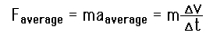the impulse of force can be extracted and found to be equal to the change in momentum of an object provided the mass is constant: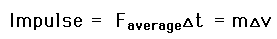Calculation

## Elastic Collisions

A perfectly elastic collision is defined as one in which there is no loss of kinetic energy in the collision.

Simply put, if objects collide without doing permanent damage, their collision can be described as elastic.

## Inelastic Collisions

An inelastic collision is one in which part of the kinetic energy is changed to some other form of energy in the collision. This usually happens by the kinetic energy being transformed into some other form of energy such as heat and/or by doing some work, such as smashing a car into a wall. Usually, collisions where there is permanent damage, or deformation, of one or more of the colliding objects are considered inelastic.

Note: Any  collision between objects will convert some of the kinetic energy into internal energy and other forms of energy, so no large scale impacts are perfectly elastic.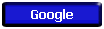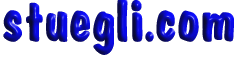Copyright © 2005 -  S. B. EglI